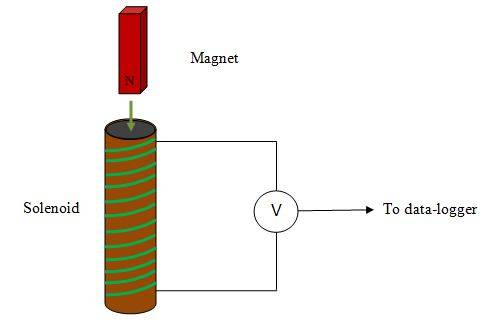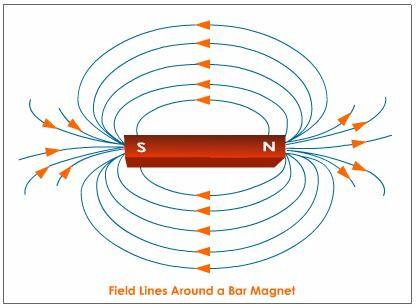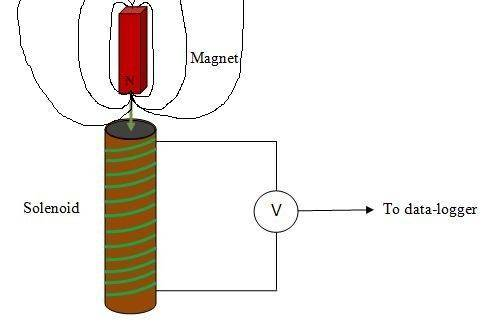# Fleming's Right Hand Rule

GeneralOJB
Can I use Fleming's right hand rule to determine the direction of current when a magnet is moved into a coil(s) of wire? I am finding it difficult because the magnetic field and movement are parallel instead of at right angles.

If not, does this mean I am only allowed to use it when a wire moves in a magnetic field (at right angles)?

dauto
Are you sure they are parallel? I don't think so.

GeneralOJB
Are you sure they are parallel? I don't think so.

This is what is confusing me then.

Consider a set-up like this:The magnet field points up (north to south) and the movement is also vertical, so the field and movement are parallel.

Gold MemberThe magnet field points up (north to south) and the movement is also vertical, so the field and movement are parallel.
Where is the south? Down? Magnetic field is from North to south.

GeneralOJB
Where is the south? Down? Magnetic field is from North to south.

The south is on the other end of the magnet, above the north.

Gold Member
The south is on the other end of the magnet, above the north.Is the field parallel to the bar?

GeneralOJBIs the field parallel to the bar?

Yes (I presume so).

GeneralOJBDo you see why it's not parallel now?

I was told that the field is from north to south, so is that not really true then? It seems the field is both perpendicular and parallel from that diagram. Also how can I use the left hand rule to determine the direction of current in this case if the field is going in infinitely many different directions perpendicular to the movement?

Gold Member
how can I use the left hand rule to determine the direction of current in this case ?
I wanted an answer to this problem too but I didn't really understand what my teacher told me.

The problems with infinite field lines seems far too advanced to me. May a more experienced member help thou and myself.
dauto!

Gold Member
(1) It's the right hand generator rule that you need. (2) Suppose the magnet to be stationary and the solenoid moving up the page in the diagram in post 8. (3) Now concentrate on just one small section of wire in the solenoid; let's choose the piece in the top front of the coil. (4) Observe that in the region of this section of wire the magnet's magnetic field is more or less coming out of the page towards you. (5) So point first finger of right hand out of the page and thumb up the page (the way the wire is moving. (6) Then your second finger will point in the direction of the induced emf. You should find that this is roughly from left to right - if you haven't dislocated any joints. (7) You'll find the same result: emf anticlockwise (looking from above) around the solenoid whichever section of wire you choose.

There's a much nicer way of finding the direction of the induced emf in cases like this: use Lenz's law… The current that will flow as a result of the emf if we short-circuit the coil (join its ends together) will produce a magnetic field which will oppose the coming together of the magnet and the solenoid. So the top end of the solenoid will become a North pole, that is flux lines will come upwards out of it. Using the right hand grip rule, we can deduce that the current, and hence the emf, in the coil is anticlockwise (seen from above).

It would be nice if you say whether this is of any help...

Last edited: